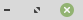https://dev.webpages.dk/PHP Order the output from a DB
PHP Order the output from a Database:To make order in a query of a database you can do exactly that, use; ORDER.
To use ORDER will sort your output. F.ex ORDER BY auto_id ASC.
The ASC will order the output in ascending order, while DESC (descending) will order the output in descending order.

### Order the output from a DB

How do you ORDER the content from a Database using PHP?

Now I will show how to ORDER the output from a query.
We order the output by choosing a field from our table that we want to order the query after. In our example database this can be for example the AutoID field, where each row is given a unique number. We can also order the listing we make by ORDER BY Lastname, or whatever we have in our database.

In this example (on the PHP tab), we ORDER BY AutoID. We can choose to order from low numbers to higher numbers (older to newer). Or we can make the listing go from high numbers to lower numbers (newer to older rows).
This is done by the ASC or DESC, as you see in the code below. If you use ASC/DESC in fields containing characters it will order from A-Z (ASC), or Z-A (DESC).

Now create a .php file and name it 'orderby.php'. Paste in the code below, and then open it in your browser.

http://127.0.0.1/orderby.php

You will see the AutoID number printet before the name of your contacts in the table we use in our examples.
Then try to change the SQL string in this example, and write ASC instead of DESC. Load the page again, and now, in the listing, you should see your contacts listed from the lowest number to the higher numbers.

### HTML

``````<HTML><BODY>
<FORM METHOD='POST' ACTION=''>
<TABLE>
<TR>
<TD>Our contacts: </TD>
``````
``````
<?php
\$con = mysqli_connect('127.0.0.1', 'root', 'Your_Password', 'dbname');

if (mysqli_connect_errno()) {
echo 'Error connecting to DB';
}

\$SQL_String = mysqli_query(\$con, 'SELECT * FROM contacts ORDER BY AutoID ASC');

while(\$row = mysqli_fetch_array(\$SQL_String))  {
echo '<OPTION VALUE=' . \$row['AutoID'] . '>' . \$row['Firstname'] . ' ' . \$row['Lastname'];
}
mysqli_close (\$con);
?>
``````
``````
</SELECT>
</TD>
</TR>
<TR>
<TD></TD>

</TR>
</TABLE>
</FORM>
</BODY></HTML>``````

### PHP

``````<?php
\$con = mysqli_connect('127.0.0.1', 'root', 'Your_Password', 'dbname');

if (mysqli_connect_errno()) {
echo 'Error connecting to DB';
}

\$SQL_String = mysqli_query(
\$con, 'SELECT * FROM contacts ORDER BY AutoID DESC');

while(\$row = mysqli_fetch_array(\$SQL_String))  {
echo '' . \$row['AutoID'] . ': ' . \$row['Firstname'] . ' ' . \$row['Lastname'];
}
mysqli_close (\$con); ?>``````